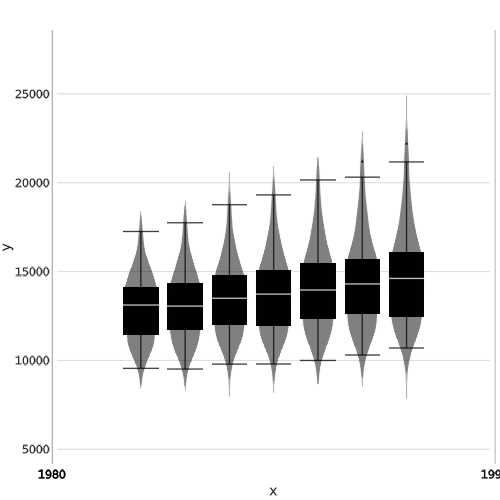# StatsMakie Tutorial

This tutorial shows how to create data visualizations using the StatsMakie grouping and styling APIs as well as the StatsMakie statistical recipes.

## Grouping data by discrete variables

The first feature that StatsMakie adds to Makie is the ability to group data by some discrete variables and use those variables to style the result. Let's first create some vectors to play with:

using Makie
using StatsMakie
using DataFrames, RDatasets
using StatsMakie: linear, smooth

N = 1000
a = rand(1:2, N) # a discrete variable
b = rand(1:2, N) # a discrete variable
x = randn(N) # a continuous variable
y = @. x * a + 0.8*randn() # a continuous variable
z = x .+ y # a continuous variable


To see how x and y relate to each other, we could simply try (be warned: the first plot is quite slow, the following ones will be much faster):



scatter(x, y, markersize = 0.2)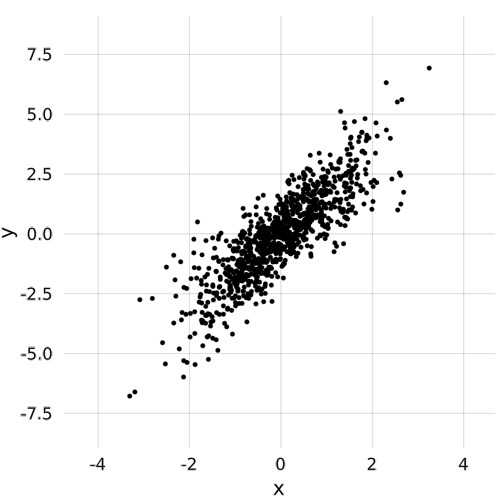It looks like there are two components in the data, and we can ask whether they come from different values of the a variable:



scatter(Group(a), x, y, markersize = 0.2)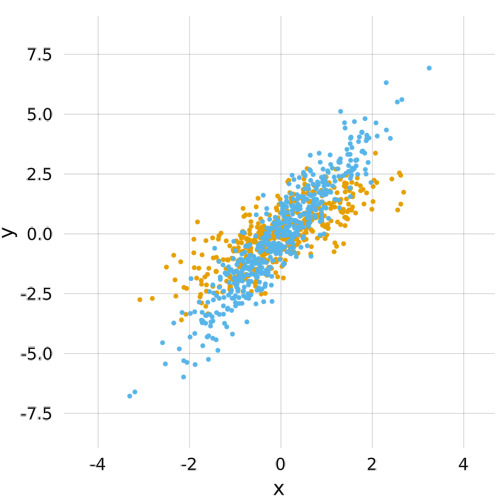Group will split the data by the discrete variable we provided and color according to that variable. Colors will cycle across a range of default values, but we can easily customize those:



scatter(Group(a), x, y, color = [:black, :red], markersize = 0.2)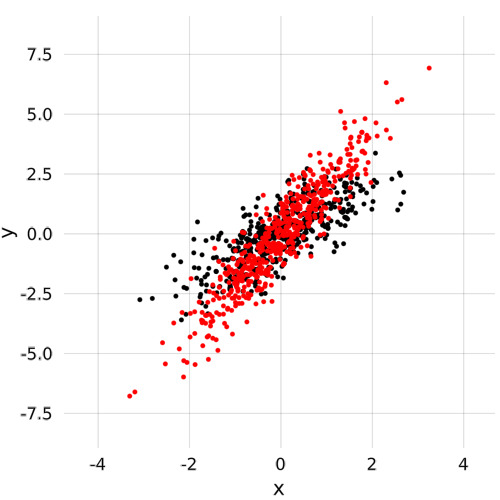and of course we are not limited to grouping with colors: we can use the shape of the marker instead. Group(a) defaults to Group(color = a), whereas Group(marker = a) with encode the information about variable a in the marker:



scatter(Group(marker = a), x, y, markersize = 0.2)Grouping by many variables is also supported:



scatter(Group(marker = a, color = b), x, y, markersize = 0.2)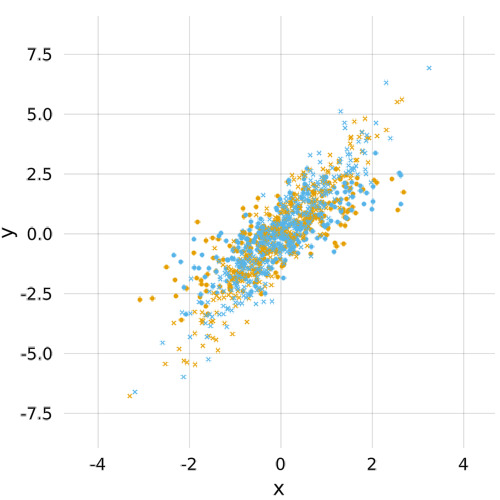## Styling data with continuous variables

One of the advantage of using an inherently discrete quantity (like the shape of the marker) to encode a discrete variable is that we can use continuous attributes (e.g. color within a colorscale) for continuous variable. In this case, if we want to see how a, x, y, z interact, we could choose the marker according to a and style the color according to z:



scatter(Group(marker = a), Style(color = z), x, y)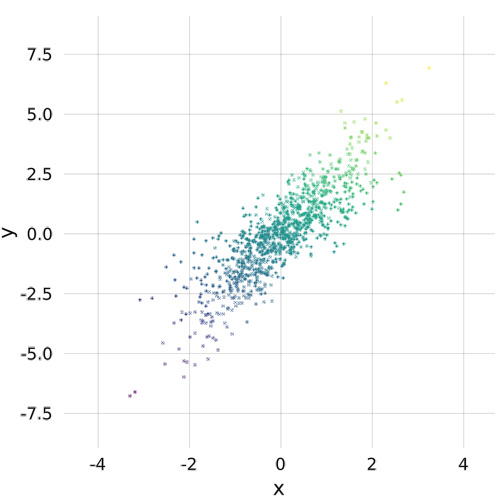Just like with Group, we can Style any number of attributes in the same plot. color is probably the most common, markersize is another sensible option (especially if we are using color already for the grouping):



scatter(Group(color = a), x, y, Style(markersize = z ./ 10))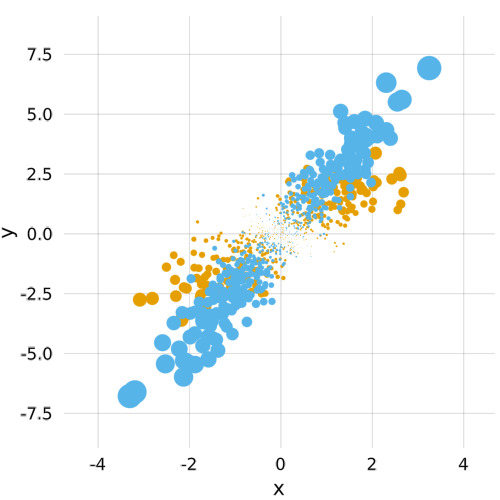## Split-apply-combine strategy with a plot

StatsMakie also has the concept of a "visualization" function (which is somewhat different but inspired on Grammar of Graphics statistics). The idea is that any function whose return type is understood by StatsMakie (meaning, there is an appropriate visualization for it) can be passed as first argument and it will be applied to the following arguments as well.

A simple example is probably linear and non-linear regression.

### Linear regression

StatsMakie knows how to compute both a linear and non-linear fit of y as a function of x, via the "analysis functions" linear (linear regression) and smooth (local polynomial regression) respectively:



plot(linear, x, y)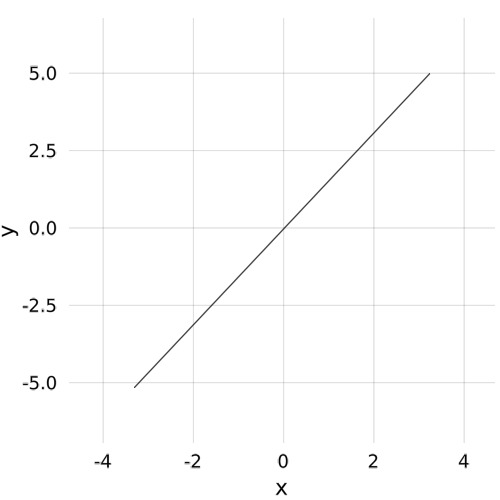That was anti-climatic! It is the linear prediction of y given x, but it's a bit of a sad plot! We can make it more colorful by splitting our data by a, and everything will work as above:



plot(linear, Group(a), x, y)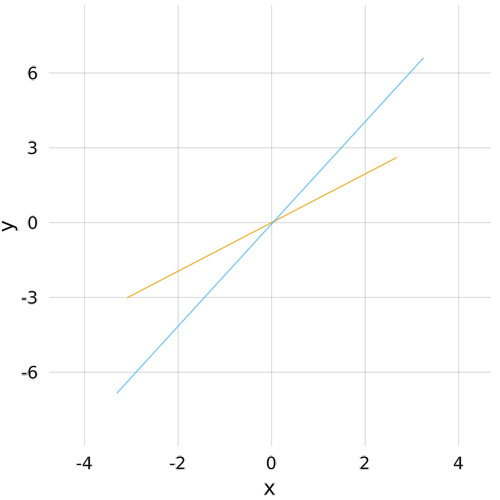And then we can plot it on top of the previous scatter plot, to make sure we got a good fit:



scatter(Group(a), x, y, markersize = 0.2)
plot!(linear, Group(a), x, y)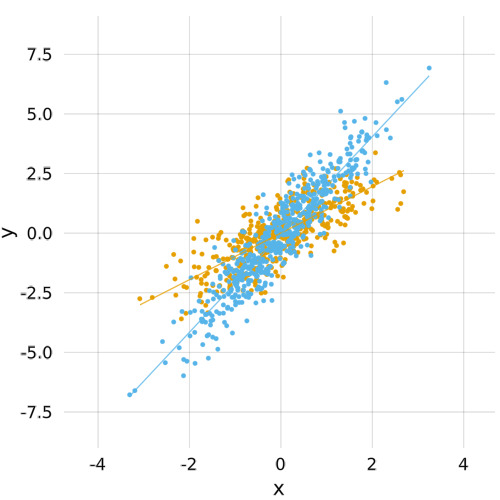Here of course it makes sense to group both things by color, but for line plots we have other options like linestyle:



plot(linear, Group(linestyle = a), x, y)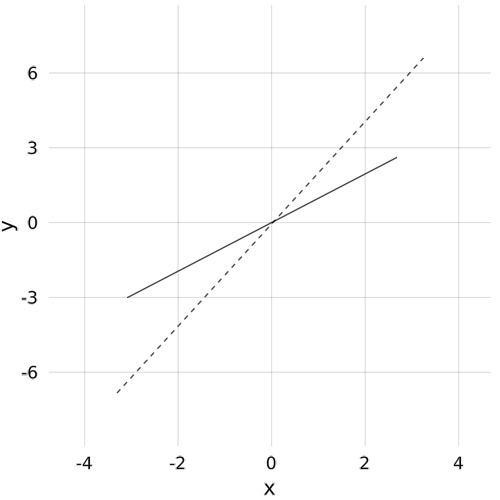### A non-linear example

Using non-linear techniques here is not very interesting as linear techniques work quite well already, so let's change variables:



plot(linear, Group(linestyle = a), x, y)and then:



N = 200
x = 10 .* rand(N)
a = rand(1:2, N)
y = sin.(x) .+ 0.5 .* rand(N) .+ cos.(x) .* a


### Different analyses

linear and smooth are two examples of possible analysis, but many more are possibles and it's easy to add new ones. If we were interested to the distributions of x and y for example we could do:



scatter(Group(a), x, y)
plot!(smooth, Group(a), x, y)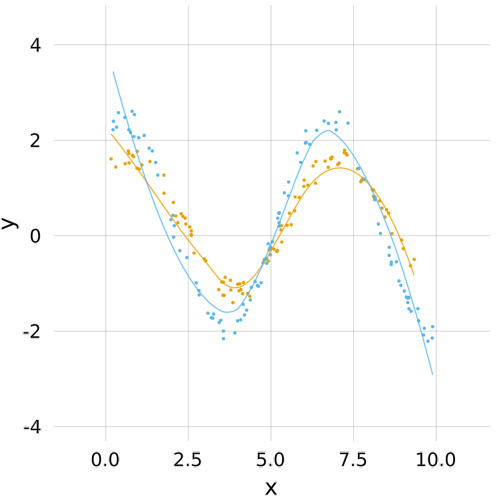The default plot type is determined by the dimensionality of the input and the analysis. A histogram analysis over one input variable produces a bar plot:



plot(histogram, y)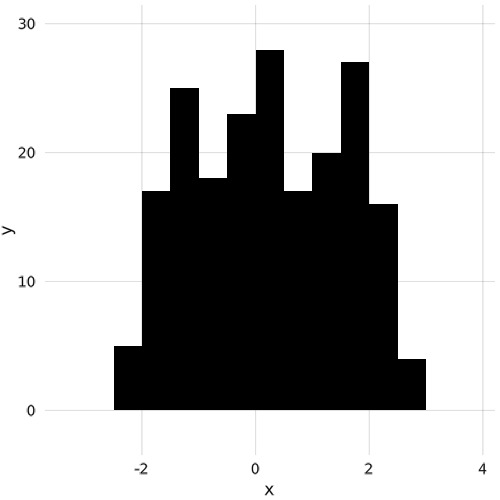whereas with two variables one would get a heatmap:



plot(histogram, x, y)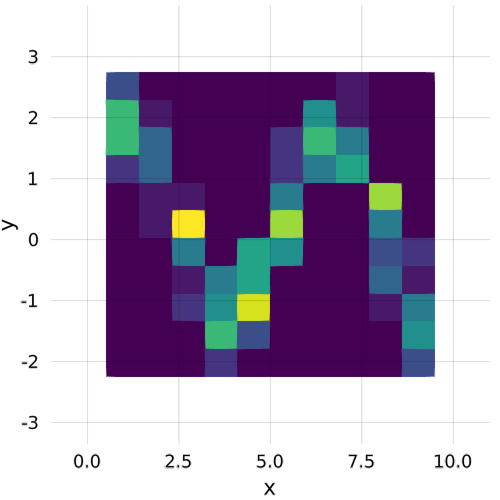This plots is reasonably customizable in that one can pass keywords arguments to the histogram analysis:



plot(histogram(nbins = 30), x, y)and change the default plot type to something else:



wireframe(histogram(nbins = 30), x, y)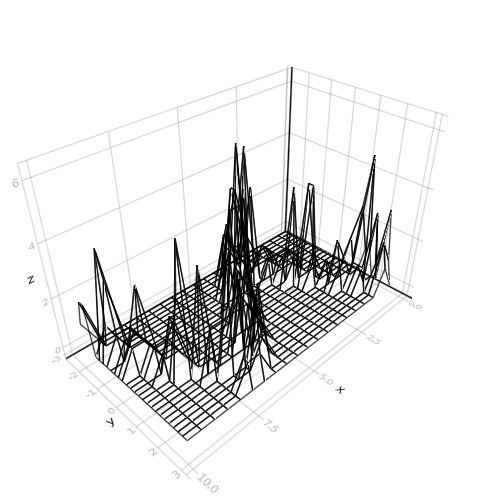Of course heatmap is the saner choice, but why not abuse Makie 3D capabilities?

Other available analysis are density (to use kernel density estimation rather than binning) and frequency (to count occurrences of discrete variables).

## What if my data is in a table, such as a DataFrame?

It is possible to signal StatsMakie that we are working from a DataFrame (or any table actually) and it will interpret symbols as columns:



iris = RDatasets.dataset("datasets", "iris")
scatter(Data(iris), Group(:Species), :SepalLength, :SepalWidth)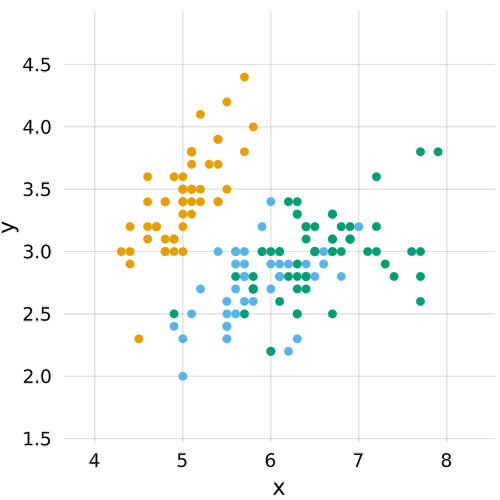And everything else works as usual:


# use Position.stack to signal that you want bars stacked vertically rather than superimposed
plot(Position.stack, histogram, Data(iris), Group(:Species), :SepalLength)wireframe(
density(trim=true),
Data(iris), Group(:Species), :SepalLength, :SepalWidth,
transparency = true, linewidth = 0.1
)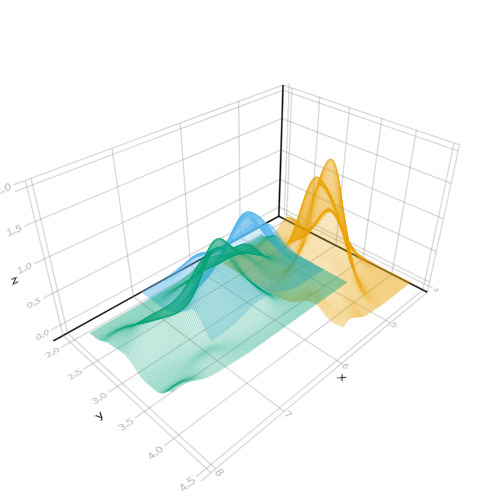### Plotting multiple columns

Other than comparing the same column split by a categorical variable, one may also compare different columns put side by side (here in a Tuple, (:PetalLength, :PetalWidth)). The attribute that styles them has to be set to bycolumn. Here color will distinguish :PetalLength versus :PetalWidth whereas the marker will distinguish the species.



scatter(
Data(iris),
Group(marker = :Species, color = bycolumn),
:SepalLength, (:PetalLength, :PetalWidth)
)## Analysis of data

There are multiple options with which to analyze your data before plotting it. These are:

• density (kernel density estimation, 1D or 2D)
• histogram (1D, 2D or even 3D!)
• frequency (count occurrences of discrete variables, 1 or 2D)
• linear (linear regression)
• smooth (LOESS regression)

To use these analyses, one can simply write something like plot(density, x, y). One can also pass options to the analysis, as in: plot(density(bandwidth=0.1), x, y), or something analogous for other analyses.

For example, see the initial setup below:

using Makie
using StatsMakie
using DataFrames, RDatasets # for data
using StatsMakie: smooth, linear
using Distributions

mtcars = dataset("datasets", "mtcars")    # load dataset of car statistics
iris = dataset("datasets", "iris")

disallowmissing!.([mtcars, iris])  # convert columns from Union{T, Missing} to T
# We can use this because the dataset has no missing values.



for which one can plot a kernel density estimation:



# kde

plot(
density,           # the type of analysis
Data(mtcars),
:MPG,
Group(color = :Cyl)
)or a histogram:



# histogram

plot(
histogram,         # the type of analysis
Data(mtcars),
:MPG,
Group(color = :Cyl)
)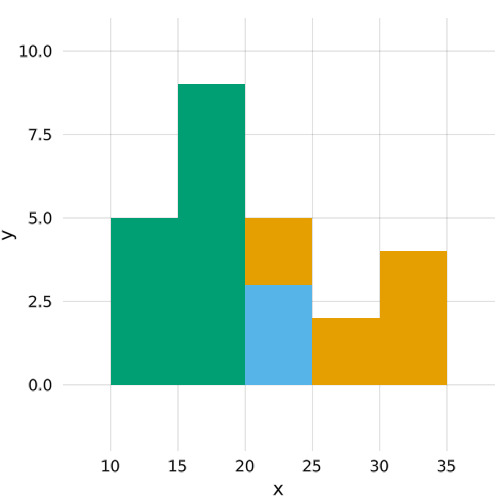One can also count the frequency of a discrete variable:



# frequency analysis

d = rand(Poisson(), 1000)
plot(frequency, d)Fitting data using LOESS fitting is of course possible:



xs = 10 .* rand(100)
ys = sin.(xs) .+ 0.5 .* rand.()
scatter(xs, ys)
plot!(smooth, xs, ys)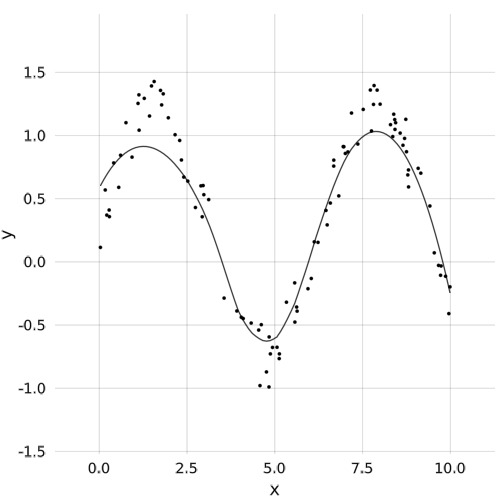and, as seen earlier, fitting it with a line is possible as well.



scatter(xs, ys)
plot!(linear, xs, ys)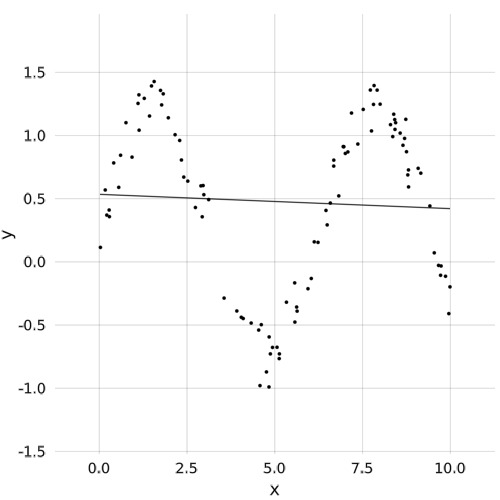## Statistical plot types

One can use box plots and violin plots with the same interface as StatsPlots.

One can create a box plot:

using Makie
using RDatasets, StatsMakie

d = dataset("Ecdat","Fatality")
boxplot(Data(d), :Year, :Perinc)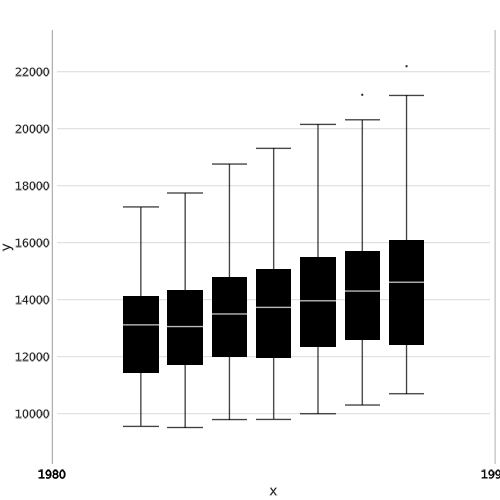or a violin plot:

using Makie
using RDatasets, StatsMakie

d = dataset("Ecdat", "Fatality");

violin(Data(d), :Year, :Perinc)and the two can be superimposed:

using Makie
using RDatasets, StatsMakie

d = dataset("Ecdat","Fatality");

violin(d.Year, d.Perinc, color = :gray)
boxplot!(d.Year, d.Perinc, color = :black)